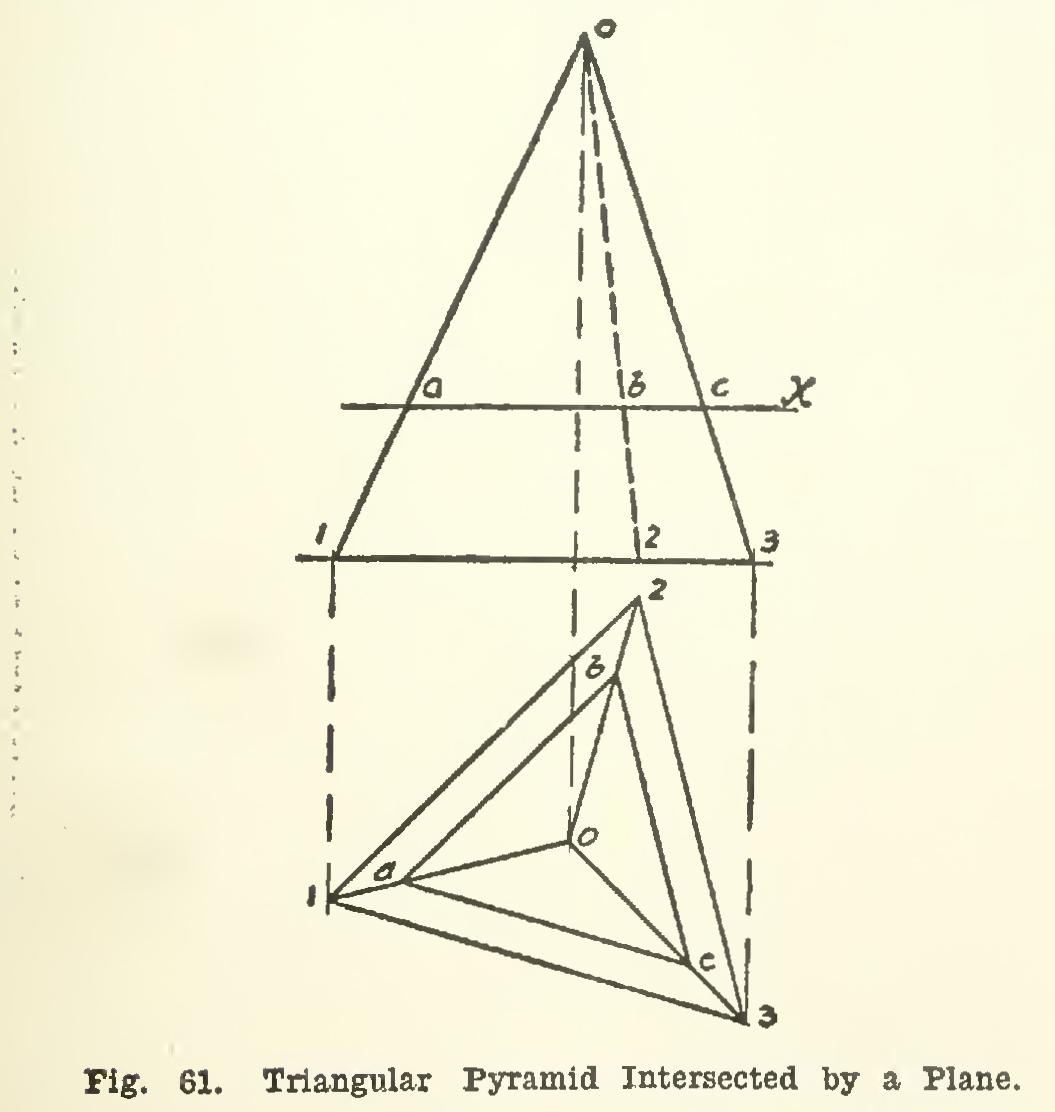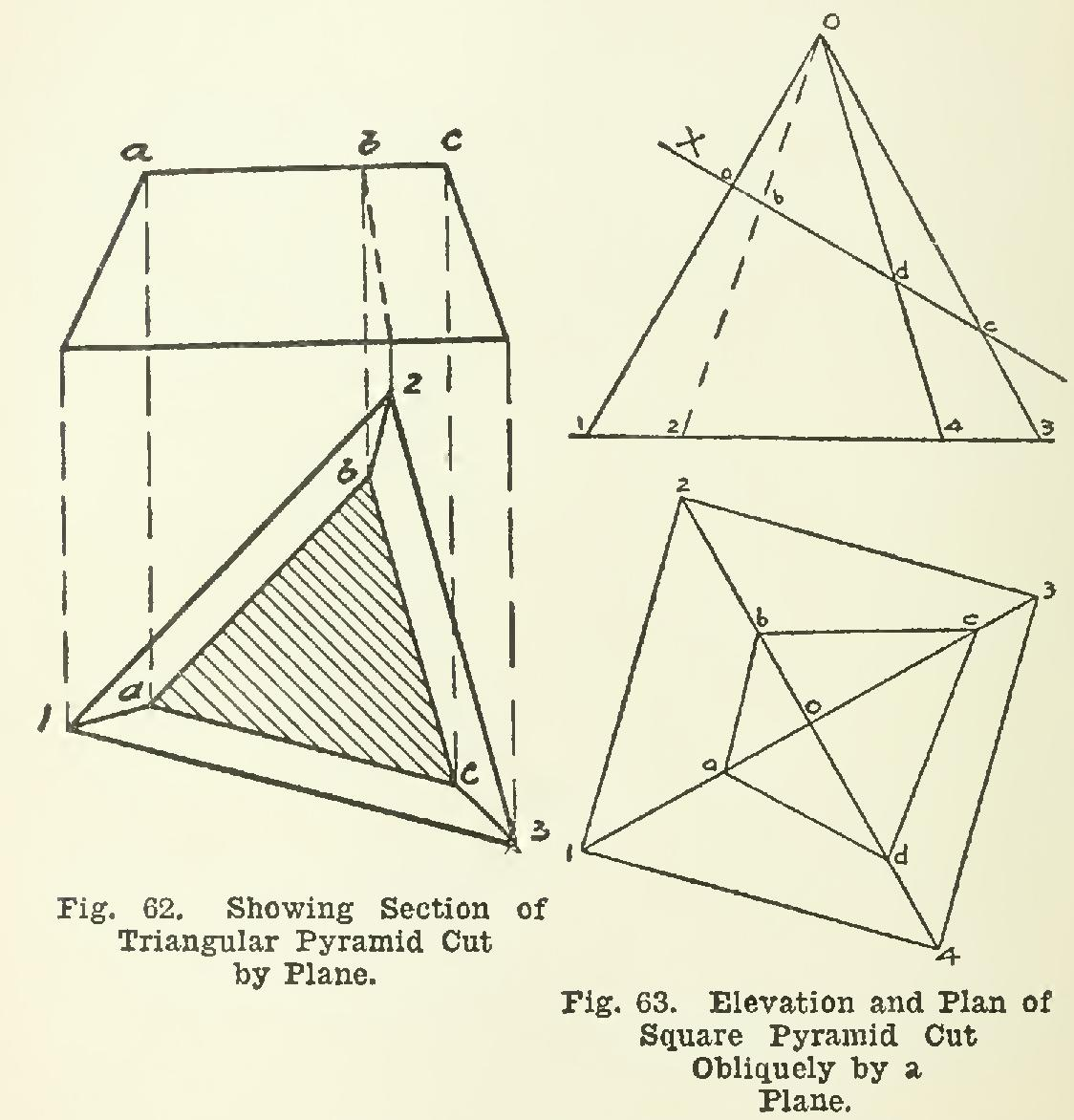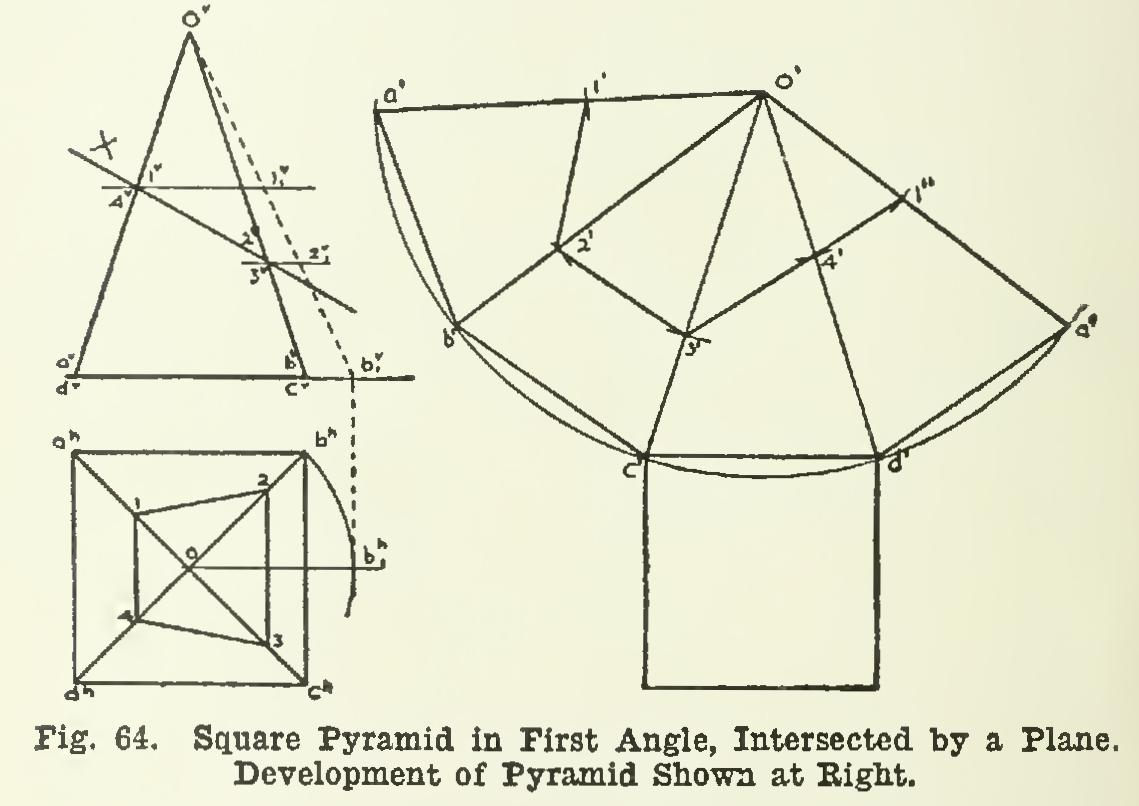Home >> Radfords-cyclopedia-of-construction-vol-1-mechanical-drafting >> Architectural Drawings to Working Drawings 134 >> Intersection and Development 75_P1

Intersection and Development 75

plane, pyramid, line, base, parallel, true and surface

Page: 1 2 3 4 5 6 7 8 9

INTERSECTION AND DEVELOPMENT.

75. Intersection.

When two surfaces meet at an angle so that if either one were extended it would actually cut the other surface, the line of meeting is called the intersection of the two surfaces.

The line of intersection may be straight or curved, according to the nature of the surface.

Planes, as the simplest kind of surfaces, will be considered first.

76. Development.

By the term development is meant a figure showing the true size and shape of the surface of any given solid, when the surface has been rolled or spread out on a plane. A true development is obtained when all of the surface is made to coincide with a plane, without overlapping or folding. Such a de velopment, when cut out of paper or thin sheet metal, and properly bent or rolled up, will reproduce the original solid.

Some surfaces are truly developable, and others are not. Among the former are all sur faces composed of planes, as those of prisms and pyramids, all cylindrical, and all conical sur faces. The most familiar solid which has a non developable surface is the sphere.

Intersection of Planes 77. The intersection of two planes is evi dently always a straight line. Familiar examples of this kind of intersection are to be found on every hand. The edge of a box is the line of intersection of two adjoining sides, which are planes; the ridgepole of a pitch roof is the line of intersection of the two sides of the roof; and so on in great number.

78. In Fig. 61, a triangular pyramid stands on its base, and several lines of intersection are shown. The slanting edges of the pyramid are the lines of intersection of the sloping faces.Let X represent a plane seen edgewise. Then X is parallel to the H plane, and perpen dicular to V. This plane cutting the pyramid will intersect all of the inclined faces, giving thereby three lines of intersection. The plane X, seen edgewise in elevation, cuts the slanting edges in points a, b, and c; and these points are found in plan directly below on the correspond ing edges.As the plane cuts edge 0-1 at a, and 0-3 at c, it will cut the face 013 in the line joining a and c; and, by joining point b with a and c, the other two lines are determined, and the whole inter section abc completed.

Two features of the intersection should be noted: First, it is a triangle. This must always be

the case when the pyramid is triangular and the intersecting plan cuts all three edges, even if the plane should not be parallel to the base.

Second, the sides of the triangle abc are re spectively parallel to the edges of the base of the pyramid. This parallel relation is due to the fact that the plane X in this case is parallel to the base.

79. It may be proved by geometry that if any prism, pyramid, cylinder, or cone is cut by a plane parallel to the base, the intersection is always a figure like the base in shape, and parallel to it in position.

80. Fig. 62 shows the same pyramid with the part above plane X removed, showing in plan the actual surface cut by the plane.

81. Fig. 63 is a square pyramid standing on its base, and cut by a plane X. The plane X in this figure is oblique to H, but the intersection abed is found by the same process as before. Notice here, that while the intersection has as many sides as the base, it is not parallel to it.

82. Fig. 64 shows a square pyramid in the first angle, intersected in a similar way by a plane X; it shows also the development of the pyramid.

Let us see how to construct the development.

Each sloping face of the pyramid is an isosceles triangle, and the base is a square; so the develop ment will consist of four equal triangles and a square, joined together and shown in their true sizes. The pyramid may be considered to be placed with its vertex at co', the edge 0-a at o'-a', and the face oab in contact with the plane of the paper. Then the triangle oab must be shown in its true size at o'a'b'.It is then necessary to find the true length of the sides of the triangle oab. The H projec tion o-bh does not show the true length of the line; neither does the V projection o∞-b∞. Let the pyramid be revolved about its axis through 45 degrees, so that o-bh will move to Then the line o-b will be parallel to V; and its pro jection on V, when found, will show the true length of the line. In revolving, the apex 0 will not move, and the point b will stay on 11; hence by, will be projected from bbl to the plane of the base or the GL, and will be the true length of the line o-b.

Page: 1 2 3 4 5 6 7 8 9